## Free Statistics

of Irreproducible Research!

Author's title
Author*The author of this computation has been verified*
R Software Modulerwasp_meanplot.wasp
Title produced by softwareMean Plot
Date of computationSat, 12 Nov 2011 10:40:31 -0500
Cite this page as followsStatistical Computations at FreeStatistics.org, Office for Research Development and Education, URL https://freestatistics.org/blog/index.php?v=date/2011/Nov/12/t1321112443sl1v1nr9u4fs8bu.htm/, Retrieved Tue, 31 Jan 2023 00:52:59 +0000
Statistical Computations at FreeStatistics.org, Office for Research Development and Education, URL https://freestatistics.org/blog/index.php?pk=141489, Retrieved Tue, 31 Jan 2023 00:52:59 +0000
QR Codes:Original text written by user:
IsPrivate?No (this computation is public)
User-defined keywords
Estimated Impact75
Family? (F = Feedback message, R = changed R code, M = changed R Module, P = changed Parameters, D = changed Data)
-     [Bivariate Data Series] [Bivariate dataset] [2008-01-05 23:51:08] [74be16979710d4c4e7c6647856088456]
F RMPD  [Mean Plot] [Colombia Coffee] [2008-01-07 13:38:24] [74be16979710d4c4e7c6647856088456]
- RM D      [Mean Plot] [Workshop 6 Simple...] [2011-11-12 15:40:31] [5c44e6aad476a1bab98fc6774eca4c08] [Current]
Feedback Forum

Post a new message
Dataseries X:
14
18
11
12
16
18
14
14
15
15
17
19
10
16
18
14
14
17
14
16
18
11
14
12
17
9
16
14
15
11
16
13
17
15
14
16
9
15
17
13
15
16
16
12
12
11
15
15
17
13
16
14
11
12
12
15
16
15
12
12
8
13
11
14
15
10
11
12
15
15
14
16
15
15
13
12
17
13
15
13
15
16
15
16
15
14
15
14
13
7
17
13
15
14
13
16
12
14
17
15
17
12
16
11
15
9
16
15
10
10
15
11
13
14
18
16
14
14
14
14
12
14
15
15
15
13
17
17
19
15
13
9
15
15
15
16
11
14
11
15
13
15
16
14
15
16
16
11
12
9
16
13
16
12
9
13
13
14
19
13
12
13

 Summary of computational transaction Raw Input view raw input (R code) Raw Output view raw output of R engine Computing time 3 seconds R Server 'Herman Ole Andreas Wold' @ wold.wessa.net

\begin{tabular}{lllllllll}
\hline
Summary of computational transaction \tabularnewline
Raw Input & view raw input (R code)  \tabularnewline
Raw Output & view raw output of R engine  \tabularnewline
Computing time & 3 seconds \tabularnewline
R Server & 'Herman Ole Andreas Wold' @ wold.wessa.net \tabularnewline
\hline
\end{tabular}
%Source: https://freestatistics.org/blog/index.php?pk=141489&T=0

[TABLE]
[ROW][C]Summary of computational transaction[/C][/ROW]
[ROW][C]Raw Input[/C][C]view raw input (R code) [/C][/ROW]
[ROW][C]Raw Output[/C][C]view raw output of R engine [/C][/ROW]
[ROW][C]Computing time[/C][C]3 seconds[/C][/ROW]
[ROW][C]R Server[/C][C]'Herman Ole Andreas Wold' @ wold.wessa.net[/C][/ROW]
[/TABLE]
Source: https://freestatistics.org/blog/index.php?pk=141489&T=0

Globally Unique Identifier (entire table): ba.freestatistics.org/blog/index.php?pk=141489&T=0

As an alternative you can also use a QR Code:

The GUIDs for individual cells are displayed in the table below:

 Summary of computational transaction Raw Input view raw input (R code) Raw Output view raw output of R engine Computing time 3 seconds R Server 'Herman Ole Andreas Wold' @ wold.wessa.net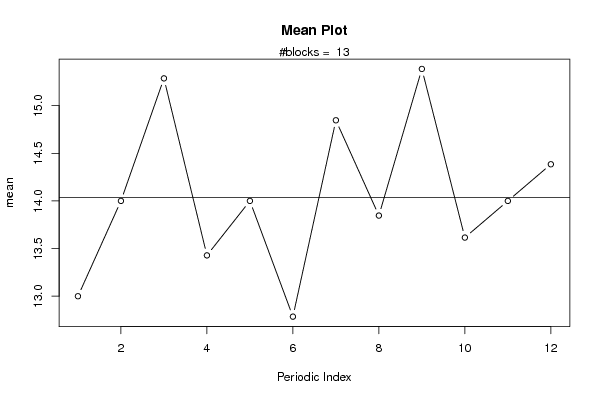PNG link Postscript link PDF link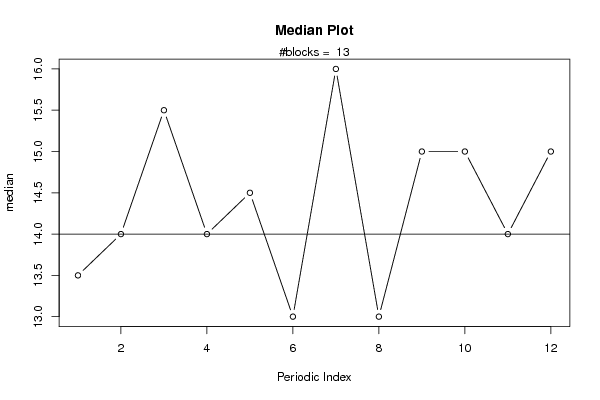PNG link Postscript link PDF link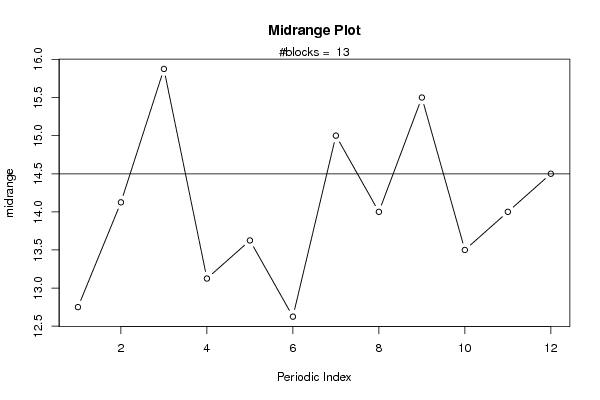PNG link Postscript link PDF link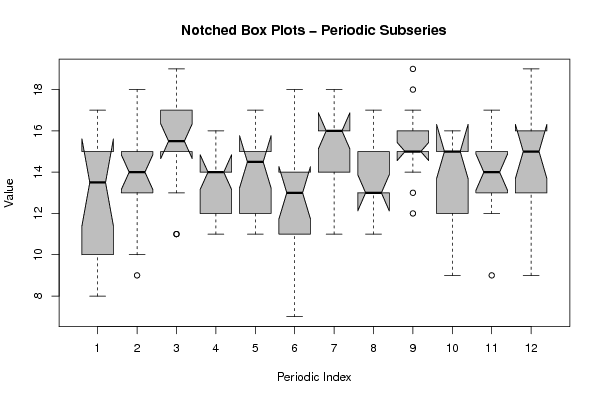PNG link Postscript link PDF link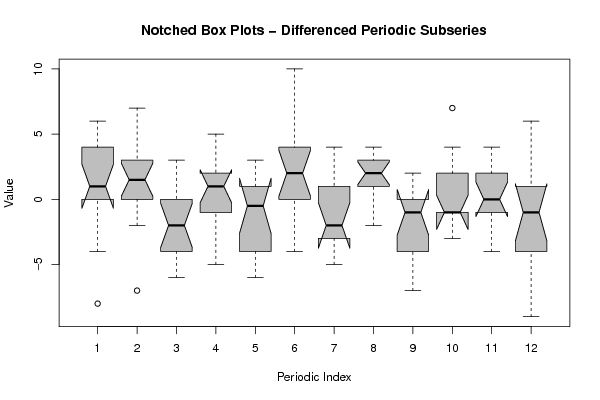PNG link Postscript link PDF link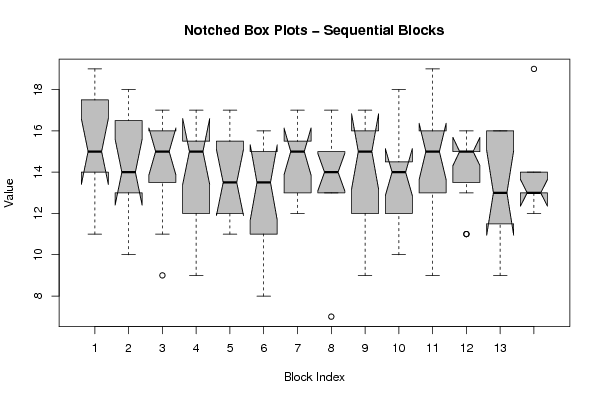PNG link Postscript link PDF link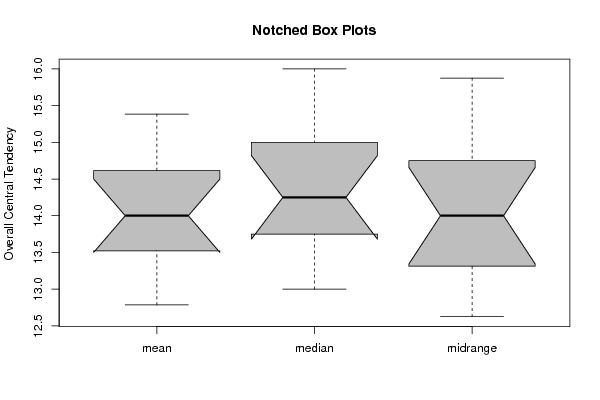PNG link Postscript link PDF link

Parameters (Session):
Parameters (R input):
par1 = 12 ;
R code (references can be found in the software module):
par1 <- as.numeric(par1)(n <- length(x))(np <- floor(n / par1))arr <- array(NA,dim=c(par1,np+1))darr <- array(NA,dim=c(par1,np+1))ari <- array(0,dim=par1)dx <- diff(x)j <- 0for (i in 1:n){j = j + 1ari[j] = ari[j] + 1arr[j,ari[j]] <- x[i]darr[j,ari[j]] <- dx[i]if (j == par1) j = 0}ariarrdarrarr.mean <- array(NA,dim=par1)arr.median <- array(NA,dim=par1)arr.midrange <- array(NA,dim=par1)for (j in 1:par1){arr.mean[j] <- mean(arr[j,],na.rm=TRUE)arr.median[j] <- median(arr[j,],na.rm=TRUE)arr.midrange[j] <- (quantile(arr[j,],0.75,na.rm=TRUE) + quantile(arr[j,],0.25,na.rm=TRUE)) / 2}overall.mean <- mean(x)overall.median <- median(x)overall.midrange <- (quantile(x,0.75) + quantile(x,0.25)) / 2bitmap(file='plot1.png')plot(arr.mean,type='b',ylab='mean',main='Mean Plot',xlab='Periodic Index')mtext(paste('#blocks = ',np))abline(overall.mean,0)dev.off()bitmap(file='plot2.png')plot(arr.median,type='b',ylab='median',main='Median Plot',xlab='Periodic Index')mtext(paste('#blocks = ',np))abline(overall.median,0)dev.off()bitmap(file='plot3.png')plot(arr.midrange,type='b',ylab='midrange',main='Midrange Plot',xlab='Periodic Index')mtext(paste('#blocks = ',np))abline(overall.midrange,0)dev.off()bitmap(file='plot4.png')z <- data.frame(t(arr))names(z) <- c(1:par1)(boxplot(z,notch=TRUE,col='grey',xlab='Periodic Index',ylab='Value',main='Notched Box Plots - Periodic Subseries'))dev.off()bitmap(file='plot4b.png')z <- data.frame(t(darr))names(z) <- c(1:par1)(boxplot(z,notch=TRUE,col='grey',xlab='Periodic Index',ylab='Value',main='Notched Box Plots - Differenced Periodic Subseries'))dev.off()bitmap(file='plot5.png')z <- data.frame(arr)names(z) <- c(1:np)(boxplot(z,notch=TRUE,col='grey',xlab='Block Index',ylab='Value',main='Notched Box Plots - Sequential Blocks'))dev.off()bitmap(file='plot6.png')z <- data.frame(cbind(arr.mean,arr.median,arr.midrange))names(z) <- list('mean','median','midrange')(boxplot(z,notch=TRUE,col='grey',ylab='Overall Central Tendency',main='Notched Box Plots'))dev.off()